# Nontrivial solutions of Hammerstein integral equations with reflections

## Abstract

Using the theory of fixed point index, we establish new results for the existence of nonzero solutions of Hammerstein integral equations with reflections. We apply our results to a first-order periodic boundary value problem with reflections.

MSC:34K10, 34B15, 34K13.

## 1 Introduction

In a recent paper, Cabada and Tojo  studied, by means of methods and results present in [2, 3], the first-order operator ${u}^{\prime }\left(t\right)+\omega u\left(-t\right)$ coupled with periodic boundary value conditions, describing the eigenvalues of the operator and providing the expression of the associated Green’s function in the nonresonant case. One motivation for studying this particular problem is that differential equations with reflection of the argument have seen growing interest along the years; see, for example, the papers [1, 413] and references therein. In , the authors provide the range of values of the real parameter ω for which the Green’s function has constant sign and apply these results to prove the existence of constant sign solutions for the nonlinear periodic problem with reflection of the argument

${u}^{\prime }\left(t\right)=h\left(t,u\left(t\right),u\left(-t\right)\right),\phantom{\rule{1em}{0ex}}t\in \left[-T,T\right],\phantom{\rule{2em}{0ex}}u\left(-T\right)=u\left(T\right).$
(1.1)

The methodology, analogous to the one utilized by Torres  in the case of ordinary differential equations, consists of two steps. First, we rewrite problem (1.1) as a Hammerstein integral equation with reflections of the type

$u\left(t\right)={\int }_{-T}^{T}k\left(t,s\right)\left[h\left(s,u\left(s\right),u\left(-s\right)\right)+mu\left(-s\right)\right]\phantom{\rule{0.2em}{0ex}}ds,\phantom{\rule{1em}{0ex}}t\in \left[-T,T\right],$

where the kernel k has constant sign. Then we make use of the well-known Guo-Krasnosel’skiĭ theorem on cone compression-expansion (see, for example, ).

In this paper, we continue the study of  and we prove new results regarding the existence of nontrivial solutions of Hammerstein integral equations with reflections of the form

$u\left(t\right)={\int }_{-T}^{T}k\left(t,s\right)g\left(s\right)f\left(s,u\left(s\right),u\left(-s\right)\right)\phantom{\rule{0.2em}{0ex}}ds,\phantom{\rule{1em}{0ex}}t\in \left[-T,T\right],$

where the kernel k is allowed to be not of constant sign. In order to do this, we extend the results of [16, 17], valid for Hammerstein integral equations without reflections, to the new context. We make use of a cone of functions that are allowed to change sign combined with the classical fixed point index for compact maps (we refer to  or  for further information). As an application of our theory we prove the existence of nontrivial solutions of the periodic problem with reflections (1.1).

## 2 The case of kernels that change sign

We begin with the case of kernels that are allowed to change sign. We impose the following conditions on k, f, g that occur in the integral equation:

$u\left(t\right)={\int }_{-T}^{T}k\left(t,s\right)g\left(s\right)f\left(s,u\left(s\right),u\left(-s\right)\right)\phantom{\rule{0.2em}{0ex}}ds=:Fu\left(t\right),$
(2.1)

where T is fixed in $\left(0,\mathrm{\infty }\right)$.

(C1) The kernel k is measurable, and for every $\tau \in \left[-T,T\right]$ we have

(C2) There exist a subinterval $\left[a,b\right]\subseteq \left[-T,T\right]$, a measurable function Φ with $\mathrm{\Phi }\ge 0$ a.e. and a constant $c=c\left(a,b\right)\in \left(0,1\right]$ such that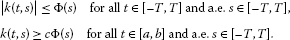(C3) The function g satisfies that $g\mathrm{\Phi }\in {L}^{1}\left[-T,T\right]$, $g\left(t\right)\ge 0$ a.e. $t\in \left[-T,T\right]$ and

${\int }_{a}^{b}\mathrm{\Phi }\left(s\right)g\left(s\right)\phantom{\rule{0.2em}{0ex}}ds>0.$

(C4) The nonlinearity $f:\left[-T,T\right]×\left(-\mathrm{\infty },\mathrm{\infty }\right)×\left(-\mathrm{\infty },\mathrm{\infty }\right)\to \left[0,\mathrm{\infty }\right)$ satisfies the Carathéodory conditions, that is, $f\left(\cdot ,u,v\right)$ is measurable for each fixed u and v and $f\left(t,\cdot ,\cdot \right)$ is continuous for a.e. $t\in \left[-T,T\right]$, and for each $r>0$, there exists ${\phi }_{r}\in {L}^{\mathrm{\infty }}\left[-T,T\right]$ such that

We recall the following definition.

Definition 2.1 Let X be a Banach Space. A cone on X is a closed, convex subset of X such that $\lambda x\in K$ for $x\in K$ and $\lambda \ge 0$ and $K\cap \left(-K\right)=\left\{0\right\}$.

Here, we work in the space $C\left[-T,T\right]$, endowed with the usual supremum norm, and we use the cone

$K=\left\{u\in C\left[-T,T\right]:\underset{t\in \left[a,b\right]}{min}u\left(t\right)\ge c\parallel u\parallel \right\}.$
(2.2)

Note that $K\ne \left\{0\right\}$.

The cone K has been essentially introduced by Infante and Webb in  and later used in [17, 1927]. K is similar to a type of cone of nonnegative functions first used by Krasnosel’skiĭ; see, e.g., , and Guo, see, e.g., . Note that functions in K are positive on the subset $\left[a,b\right]$ but are allowed to change sign in $\left[-T,T\right]$.

We require some knowledge of the classical fixed point index for compact maps; see for example  or  for further information. If Ω is a bounded open subset of K (in the relative topology) we denote by $\overline{\mathrm{\Omega }}$ and Ω the closure and the boundary relative to K. When D is an open bounded subset of X, we write ${D}_{K}=D\cap K$, an open subset of K.

The next lemma is a direct consequence of classical results from degree theory, for details see .

Lemma 2.2 Let D be an open bounded set with ${D}_{K}\ne \mathrm{\varnothing }$ and ${\overline{D}}_{K}\ne K$. Assume that $F:{\overline{D}}_{K}\to K$ is a compact map such that $x\ne Fx$ for $x\in \partial {D}_{K}$. Then the fixed point index ${i}_{K}\left(F,{D}_{K}\right)$ has the following properties:

1. (1)

If there exists $e\in K\setminus \left\{0\right\}$ such that $x\ne Fx+\lambda e$ for all $x\in \partial {D}_{K}$ and all $\lambda >0$, then ${i}_{K}\left(F,{D}_{K}\right)=0$.

2. (2)

If $\mu x\ne Fx$ for all $x\in \partial {D}_{K}$ and for every $\mu \ge 1$, then ${i}_{K}\left(F,{D}_{K}\right)=1$.

3. (3)

Let ${D}^{1}$ be open in X with $\overline{{D}^{1}}\subset {D}_{K}$. If ${i}_{K}\left(F,{D}_{K}\right)=1$ and ${i}_{K}\left(F,{D}_{K}^{1}\right)=0$, then F has a fixed point in ${D}_{K}\setminus \overline{{D}_{K}^{1}}$. The same result holds if ${i}_{K}\left(F,{D}_{K}\right)=0$ and ${i}_{K}\left(F,{D}_{K}^{1}\right)=1$.

Definition 2.3 We use the following sets:

${K}_{\rho }=\left\{u\in K:\parallel u\parallel <\rho \right\},\phantom{\rule{2em}{0ex}}{V}_{\rho }=\left\{u\in K:\underset{t\in \left[a,b\right]}{min}u\left(t\right)<\rho \right\}.$

The set ${V}_{\rho }$ was introduced in  and is equal to the set called ${\mathrm{\Omega }}_{\rho /c}$ in . The notation ${V}_{\rho }$ makes it clear that choosing c as large as possible yields a weaker condition to be satisfied by f in Lemma 2.6. A key feature of these sets is that they can be nested, that is,

${K}_{\rho }\subset {V}_{\rho }\subset {K}_{\rho /c}.$

Theorem 2.4 Assume that hypotheses (C1)-(C4) hold for some $r>0$. Then F maps ${\overline{K}}_{r}$ into K and is compact. When these hypotheses hold for each $r>0$, F is compact and maps K into K.

Proof For $u\in {\overline{K}}_{r}$ and $t\in \left[-T,T\right]$, we have

$\begin{array}{rcl}|Fu\left(t\right)|& \le & {\int }_{-T}^{T}|k\left(t,s\right)|g\left(s\right)f\left(s,u\left(s\right),u\left(-s\right)\right)\phantom{\rule{0.2em}{0ex}}ds\\ \le & {\int }_{-T}^{T}\mathrm{\Phi }\left(s\right)g\left(s\right)f\left(s,u\left(s\right),u\left(-s\right)\right)\phantom{\rule{0.2em}{0ex}}ds\end{array}$

and

$\underset{t\in \left[a,b\right]}{min}Fu\left(t\right)\ge c{\int }_{-T}^{T}\mathrm{\Phi }\left(s\right)g\left(s\right)f\left(s,u\left(s\right),u\left(-s\right)\right)\phantom{\rule{0.2em}{0ex}}ds\ge c\parallel Fu\parallel .$

Therefore, we have that $Fu\in K$ for every $u\in {\overline{K}}_{r}$.

The compactness of F follows from the fact that the Hammerstein integral operator that occurs in (2.1) is compact (this a consequence of Proposition 3.1 of Chapter 5 of ). □

In the sequel, we give a condition that ensures that, for a suitable $\rho >0$, the index is 1 on ${K}_{\rho }$.

Lemma 2.5 Assume that

(${\mathrm{I}}_{\rho }^{1}$) there exists $\rho >0$ such that

$\frac{{f}^{-\rho ,\rho }}{m}<1,$

where

$\frac{1}{m}:=\underset{t\in \left[-T,T\right]}{sup}{\int }_{-T}^{T}|k\left(t,s\right)|g\left(s\right)\phantom{\rule{0.2em}{0ex}}ds$

and

${f}^{-\rho ,\rho }=sup\left\{\frac{f\left(t,u,v\right)}{\rho }:\left(t,u,v\right)\in \left[-T,T\right]×\left[-\rho ,\rho \right]×\left[-\rho ,\rho \right]\right\}.$

Then the fixed point index, ${i}_{K}\left(F,{K}_{\rho }\right)$, is equal to 1.

Proof We show that $\mu u\ne Fu$ for every $u\in \partial {K}_{\rho }$ and for every $\mu \ge 1$. In fact, if this does not happen, there exist $\mu \ge 1$ and $u\in \partial {K}_{\rho }$ such that $\mu u=Fu$, that is,

$\mu u\left(t\right)={\int }_{-T}^{T}k\left(t,s\right)g\left(s\right)f\left(s,u\left(s\right),u\left(-s\right)\right)\phantom{\rule{0.2em}{0ex}}ds.$

Taking the absolute value and then the supremum for $t\in \left[-T,T\right]$ gives

$\begin{array}{rcl}\mu \rho & \le & \underset{t\in \left[-T,T\right]}{sup}{\int }_{-T}^{T}|k\left(t,s\right)|g\left(s\right)f\left(s,u\left(s\right),u\left(-s\right)\right)\phantom{\rule{0.2em}{0ex}}ds\\ \le & \rho {f}^{-\rho ,\rho }\cdot \underset{t\in \left[-T,T\right]}{sup}{\int }_{-T}^{T}|k\left(t,s\right)|g\left(s\right)\phantom{\rule{0.2em}{0ex}}ds<\rho .\end{array}$

This contradicts the fact that $\mu \ge 1$ and proves the result. □

Let us see now a condition that guarantees the index is equal to zero on ${V}_{\rho }$ for some appropriate $\rho >0$.

Lemma 2.6 Assume that

(${\mathrm{I}}_{\rho }^{0}$) there exist $\rho >0$ such that such that

$\frac{{f}_{\left(\rho ,\rho /c\right)}}{M\left(a,b\right)}>1,$

where

$\frac{1}{M\left(a,b\right)}:=\underset{t\in \left[a,b\right]}{inf}{\int }_{a}^{b}k\left(t,s\right)g\left(s\right)\phantom{\rule{0.2em}{0ex}}ds$

and

${f}_{\left(\rho ,\rho /c\right)}=inf\left\{\frac{f\left(t,u,v\right)}{\rho }:\left(t,u,v\right)\in \left[a,b\right]×\left[\rho ,\rho /c\right]×\left[-\rho /c,\rho /c\right]\right\}.$

Then ${i}_{K}\left(F,{V}_{\rho }\right)=0$.

Proof Let $e\left(t\right)\equiv 1$, then $e\in K$. We prove that

In fact, if not, there exist $u\in \partial {V}_{\rho }$ and $\lambda \ge 0$ such that $u=Fu+\lambda e$. Then we have

$u\left(t\right)={\int }_{-T}^{T}k\left(t,s\right)g\left(s\right)f\left(s,u\left(s\right),u\left(-s\right)\right)\phantom{\rule{0.2em}{0ex}}ds+\lambda .$

Thus we get, for $t\in \left[a,b\right]$,

$\begin{array}{rcl}u\left(t\right)& =& {\int }_{-T}^{T}k\left(t,s\right)g\left(s\right)f\left(s,u\left(s\right),u\left(-s\right)\right)\phantom{\rule{0.2em}{0ex}}ds+\lambda \ge {\int }_{a}^{b}k\left(t,s\right)g\left(s\right)f\left(s,u\left(s\right),u\left(-s\right)\right)\phantom{\rule{0.2em}{0ex}}ds\\ \ge & \rho {f}_{\left(\rho ,\rho /c\right)}\left({\int }_{a}^{b}k\left(t,s\right)g\left(s\right)\phantom{\rule{0.2em}{0ex}}ds\right).\end{array}$

Taking the minimum over $\left[a,b\right]$ gives $\rho >\rho$ a contradiction. □

The above lemmas can be combined to prove the following theorem. Here, we deal with the existence of at least one, two or three solutions. We stress that, by expanding the lists in conditions (S5), (S6) below, it is possible to state results for four or more positive solutions; see for example the paper by Lan  for the type of results that might be stated. We omit the proof which follows directly from the properties of the fixed point index stated in Lemma 2.2(3).

Theorem 2.7 The integral equation (2.1) has at least one nonzero solution in K if either of the following conditions hold:

(S1) There exist ${\rho }_{1},{\rho }_{2}\in \left(0,\mathrm{\infty }\right)$ with ${\rho }_{1}/c<{\rho }_{2}$ such that (${\mathrm{I}}_{{\rho }_{1}}^{0}$) and (${\mathrm{I}}_{{\rho }_{2}}^{1}$) hold:

(S2) There exist ${\rho }_{1},{\rho }_{2}\in \left(0,\mathrm{\infty }\right)$ with ${\rho }_{1}<{\rho }_{2}$ such that (${\mathrm{I}}_{{\rho }_{1}}^{1}$) and (${\mathrm{I}}_{{\rho }_{2}}^{0}$) hold.

The integral equation (2.1) has at least two nonzero solutions in K if one of the following conditions hold:

(S3) There exist ${\rho }_{1},{\rho }_{2},{\rho }_{3}\in \left(0,\mathrm{\infty }\right)$ with ${\rho }_{1}/c<{\rho }_{2}<{\rho }_{3}$ such that (${\mathrm{I}}_{{\rho }_{1}}^{0}$), (${\mathrm{I}}_{{\rho }_{2}}^{1}$) and (${\mathrm{I}}_{{\rho }_{3}}^{0}$) hold.

(S4) There exist ${\rho }_{1},{\rho }_{2},{\rho }_{3}\in \left(0,\mathrm{\infty }\right)$ with ${\rho }_{1}<{\rho }_{2}$ and ${\rho }_{2}/c<{\rho }_{3}$ such that (${\mathrm{I}}_{{\rho }_{1}}^{1}$), (${\mathrm{I}}_{{\rho }_{2}}^{0}$) and (${\mathrm{I}}_{{\rho }_{3}}^{1}$) hold.

The integral equation (2.1) has at least three nonzero solutions in K if one of the following conditions hold:

(S5) There exist ${\rho }_{1},{\rho }_{2},{\rho }_{3},{\rho }_{4}\in \left(0,\mathrm{\infty }\right)$ with ${\rho }_{1}/c<{\rho }_{2}<{\rho }_{3}$ and ${\rho }_{3}/c<{\rho }_{4}$ such that (${\mathrm{I}}_{{\rho }_{1}}^{0}$), (${\mathrm{I}}_{{\rho }_{2}}^{1}$), (${\mathrm{I}}_{{\rho }_{3}}^{0}$) and (${\mathrm{I}}_{{\rho }_{4}}^{1}$) hold.

(S6) There exist ${\rho }_{1},{\rho }_{2},{\rho }_{3},{\rho }_{4}\in \left(0,\mathrm{\infty }\right)$ with ${\rho }_{1}<{\rho }_{2}$ and ${\rho }_{2}/c<{\rho }_{3}<{\rho }_{4}$ such that (${\mathrm{I}}_{{\rho }_{1}}^{1}$), (${\mathrm{I}}_{{\rho }_{2}}^{0}$), (${\mathrm{I}}_{{\rho }_{3}}^{1}$) and (${\mathrm{I}}_{{\rho }_{4}}^{0}$) hold.

## 3 The case of nonnegative kernels

We now assume the functions k, f, g that occur in (2.1) satisfy the conditions (C1)-(C4) in the previous section, where (C2) and (C4) are replaced with the following.

(${\mathrm{C}}_{2}^{\prime }$) The kernel k is nonnegative for $t\in \left[-T,T\right]$ and a.e. $s\in \left[-T,T\right]$ and there exist a subinterval $\left[a,b\right]\subseteq \left[-T,T\right]$, a measurable function Φ, and a constant $c=c\left(a,b\right)\in \left(0,1\right]$ such that(${\mathrm{C}}_{4}^{\prime }$) The nonlinearity $f:\left[-T,T\right]×\left[0,\mathrm{\infty }\right)×\left[0,\mathrm{\infty }\right)\to \left[0,\mathrm{\infty }\right)$ satisfies Carathéodory conditions, that is, $f\left(\cdot ,u,v\right)$ is measurable for each fixed u and v and $f\left(t,\cdot ,\cdot \right)$ is continuous for a.e. $t\in \left[-T,T\right]$, and for each $r>0$, there exists ${\phi }_{r}\in {L}^{\mathrm{\infty }}\left[-T,T\right]$ such that

These hypotheses enable us to work in the cone of nonnegative functions

${K}^{\prime }=\left\{u\in C\left[-T,T\right]:u\ge 0,\underset{t\in \left[a,b\right]}{min}u\left(t\right)\ge c\parallel u\parallel \right\},$
(3.1)

that is smaller than the cone (2.2). It is possible to show that F is compact and leaves the cone ${K}^{\prime }$ invariant. The conditions on the index are given by the following lemmas; the proofs are omitted as they are similar to the ones in the previous section.

Lemma 3.1 Assume that

($\overline{{\mathrm{I}}_{\rho }^{1}}$) there exists $\rho >0$ such that ${f}^{0,\rho }, where

${f}^{0,\rho }=sup\left\{\frac{f\left(t,u,v\right)}{\rho }:\left(t,u,v\right)\in \left[-T,T\right]×\left[0,\rho \right]×\left[0,\rho \right]\right\}.$

Then ${i}_{K}\left(F,{K}_{\rho }\right)=1$.

Lemma 3.2 Assume that

($\overline{{\mathrm{I}}_{\rho }^{0}}$) there exist $\rho >0$ such that ${f}_{{\left(\rho ,\rho /c\right)}^{\prime }}>M$, where

${f}_{{\left(\rho ,\rho /c\right)}^{\prime }}=inf\left\{\frac{f\left(t,u,v\right)}{\rho }:\left(t,u,v\right)\in \left[a,b\right]×\left[\rho ,\rho /c\right]×\left[0,\rho /c\right]\right\}.$

Then ${i}_{K}\left(F,{V}_{\rho }\right)=0$.

A result equivalent to Theorem 2.7 is clearly valid in this case, with nontrivial solutions belonging to the cone (3.1).

## 4 The case of kernels with extra positivity

We now assume the functions k, f, g that occur in (2.1) satisfy the conditions (C1), (${\mathrm{C}}_{2}^{\prime }$), (C3) and (${\mathrm{C}}_{4}^{\prime }$) with $\left[a,b\right]=\left[-T,T\right]$; in particular note that the kernel satisfies the stronger positivity requirement

These hypotheses enable us to work in the cone(4.1)

Remark 4.1 Note that a function in ${K}^{″}$ that possesses a nontrivial norm, has the useful property that is strictly positive on $\left[-T,T\right]$.

Once again F is compact and leaves the cone ${K}^{″}$ invariant. The assumptions on the index are as follows.

Lemma 4.2 Assume that

($\stackrel{˜}{{\mathrm{I}}_{\rho }^{1}}$) there exists $\rho >0$ such that ${f}^{c\rho ,\rho }, where

${f}^{c\rho ,\rho }=sup\left\{\frac{f\left(t,u,v\right)}{\rho }:\left(t,u,v\right)\in \left[-T,T\right]×\left[c\rho ,\rho \right]×\left[c\rho ,\rho \right]\right\}.$

Then ${i}_{K}\left(F,{K}_{\rho }\right)=1$.

Lemma 4.3 Assume that

($\stackrel{˜}{{\mathrm{I}}_{\rho }^{1}}$) there exist $\rho >0$ such that, where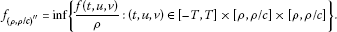Then ${i}_{K}\left(F,{V}_{\rho }\right)=0$.

A result similar to Theorem 2.7 holds in this case.

Remark 4.4 If f is defined only on $\left[-T,T\right]×\left[{u}_{1},{u}_{2}\right]×\left[{v}_{1},{v}_{2}\right]$ we can extend it to $\left[-T,T\right]×\mathbb{R}×\mathbb{R}$ considering firstly

and secondly

This approach that follows the one of Lan  and that has been exploited in  in the context of problems without reflections, is useful to prove the existence of multiple positive solutions in presence of strong singularities in the nonlinearity f. For a related result, that uses the principal eigenvalue of the corresponding linearized equation, see .

Remark 4.5 Note that results similar to Sections 2, 3 and 4 hold when the kernel k is negative on a strip, negative and strictly negative. This gives nontrivial solutions that are negative on an interval, negative and strictly negative, respectively.

## 5 An application

We now turn our attention to the first-order functional periodic boundary value problem(5.1)(5.2)

We apply the shift argument of  (a similar idea has been used in [14, 36]), by fixing $\omega \in \mathbb{R}\setminus \left\{0\right\}$ and considering the equivalent expression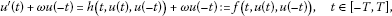(5.3)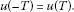(5.4)

Following the ideas developed in , we can verify that the functional boundary value problem (5.3)-(5.4) can be rewritten into a Hammerstein integral equation of the type

$u\left(t\right)={\int }_{-T}^{T}k\left(t,s\right)f\left(s,u\left(s\right),u\left(-s\right)\right)\phantom{\rule{0.2em}{0ex}}ds.$
(5.5)

Also, $k\left(t,s\right)$ can be expressed in the following way (see  for details):

(5.6)

The results that follow are meant to prove that we are under the hypothesis of Theorem 2.4.

The sign properties of the kernel (5.6) can be summarized as follows.

Theorem 5.1 

Let $\zeta =\omega T$.

1. (1)

If $\zeta \in \left(0,\frac{\pi }{4}\right)$ then $k\left(t,s\right)$ is strictly positive on ${\left[-T,T\right]}^{2}$.

2. (2)

If $\zeta \in \left(-\frac{\pi }{4},0\right)$ then $k\left(t,s\right)$ is strictly negative on ${\left[-T,T\right]}^{2}$.

3. (3)

If $\zeta =\frac{\pi }{4}$ then $k\left(t,s\right)$ vanishes on $P:=\left\{\left(-T,-T\right),\left(0,0\right),\left(T,T\right),\left(T,-T\right)\right\}$ and is strictly positive on $\left({\left[-T,T\right]}^{2}\right)\setminus P$.

4. (4)

If $\zeta =-\frac{\pi }{4}$ then $k\left(t,s\right)$ vanishes on P and is strictly negative on $\left({\left[-T,T\right]}^{2}\right)\setminus P$.

5. (5)

If $\zeta \in \mathbb{R}\setminus \left[-\frac{\pi }{4},\frac{\pi }{4}\right]$ then $k\left(t,s\right)$ is changes sign on ${\left[-T,T\right]}^{2}$.

In , some existence results has been obtained for problem (5.3)-(5.4) when $\zeta \in \left[-\frac{\pi }{4},\frac{\pi }{4}\right]$, i.e., when the kernel k has constant sign on ${\left[-T,T\right]}^{2}$. But nothing is obtained for the changing sign case. Still, there are some things to be said about the kernel k when $\zeta \in \mathbb{R}\setminus \left[-\frac{\pi }{4},\frac{\pi }{4}\right]$. First, realize that, using the trigonometric identities $cos\left(a-b\right)±sin\left(a+b\right)=\left(cosa±sina\right)\left(cosb±sinb\right)$ and $cos\left(a\right)+sin\left(a\right)=\sqrt{2}cos\left(a-\frac{\pi }{4}\right)$ and making the change of variables $t=Tz$, $s=Ty$, we can express k as

(5.7)

The following lemma relates the sign of k for ζ positive and negative.

Lemma 5.2 

${k}_{\zeta }\left(t,s\right)=-{k}_{-\zeta }\left(-t,-s\right)$ $\mathrm{\forall }t,s\in I$ where ${k}_{\zeta }$ is the kernel for the value ζ.

Now we have the following result.

Lemma 5.3 The following hold:

1. (1)

If $\zeta \in \left(\frac{\pi }{4},\frac{\pi }{2}\right)$, then k is strictly positive in

$S:=\left[\left(-\frac{\pi }{4|\zeta |},\frac{\pi }{4|\zeta |}-1\right)\cup \left(1-\frac{\pi }{4|\zeta |},\frac{\pi }{4|\zeta |}\right)\right]×\left[-1,1\right].$
2. (2)

If $\zeta \in \left(-\frac{\pi }{2},-\frac{\pi }{4}\right)$, k is strictly negative in S.

Proof By Lemma 5.2, it is enough to prove that k is strictly positive in S for $\zeta \in \left(\frac{\pi }{4},\frac{\pi }{2}\right)$. We do here the proof for the connected component $\left(1-\frac{\pi }{4\zeta },\frac{\pi }{4\zeta }\right)×\left[-1,1\right]$ of S. For the other one, the proof is analogous.

If $z\in \left(1-\frac{\pi }{4\zeta },\frac{\pi }{4\zeta }\right)$, then $\zeta z+\frac{\pi }{4}\in \left(\zeta ,\frac{\pi }{2}\right)\subset \left(\frac{\pi }{4},\frac{\pi }{2}\right)$, and hence $cos\left(\zeta z+\frac{\pi }{4}\right)>0$.

Also, if $z\in \left(1-\frac{\pi }{4\zeta },\frac{\pi }{4\zeta }\right)$, then $\zeta \left(1-z\right)-\frac{\pi }{4}\in \left(\zeta -\frac{\pi }{2},0\right)\subset \left(-\frac{\pi }{4},0\right)$ and, therefore, $cos\left(\zeta \left(1-z\right)-\frac{\pi }{4}\right)>0$.

If $y\in \left(-\frac{\pi }{4\zeta },\frac{\pi }{4\zeta }\right)$, then $\zeta y-\frac{\pi }{4}\in \left(-\frac{\pi }{2},0\right)$ so $cos\left(\zeta y-\frac{\pi }{4}\right)>0$.

If $y\in \left(1-\frac{\pi }{4\zeta },1\right)$, then $\zeta \left(y-1\right)-\frac{\pi }{4}\in \left(-\frac{\pi }{2},-\frac{\pi }{4}\right)$ so $cos\left(\zeta \left(y-1\right)-\frac{\pi }{4}\right)>0$.

If $y\in \left(-1,\frac{\pi }{4\zeta }-1\right)$, then $\zeta \left(y+1\right)+\frac{\pi }{4}\in \left(\frac{\pi }{4},\frac{\pi }{2}\right)$ so $cos\left(\zeta \left(y+1\right)+\frac{\pi }{4}\right)>0$.

With these inequalities the result is straightforward from equation (5.7). □

Lemma 5.4 If $\zeta \in \left(\frac{\pi }{4},\frac{\pi }{2}\right)$ then $sin\left(\zeta \right)|k\left(z,y\right)|\le \mathrm{\Phi }\left(y\right):=sin\left(\zeta \right){max}_{z\in \left[-1,1\right]}k\left(z,y\right)$ where Φ admits the following expression

$\mathrm{\Phi }\left(y\right)=\left\{\begin{array}{cc}cos\left[\zeta \left(y-1\right)-\frac{\pi }{4}\right]\hfill & \mathit{\text{if}}\phantom{\rule{0.5em}{0ex}}y\in \left[\beta ,1\right],\hfill \\ cos\left[\zeta \left(y-1\right)+\frac{\pi }{4}\right]cos\left(\zeta y-\frac{\pi }{4}\right)\hfill & \mathit{\text{if}}\phantom{\rule{0.5em}{0ex}}y\in \left[1-\frac{\pi }{4\zeta },\beta \right),\hfill \\ cos\left(\zeta y-\frac{\pi }{4}\right)\hfill & \mathit{\text{if}}\phantom{\rule{0.5em}{0ex}}y\in \left[\beta -1,1-\frac{\pi }{4\zeta }\right),\hfill \\ cos\left(\zeta y+\frac{\pi }{4}\right)cos\left[\zeta \left(y+1\right)-\frac{\pi }{4}\right]\hfill & \mathit{\text{if}}\phantom{\rule{0.5em}{0ex}}y\in \left[-\frac{\pi }{4\zeta },\beta -1\right),\hfill \\ cos\left[\zeta \left(y+1\right)-\frac{\pi }{4}\right]\hfill & \mathit{\text{if}}\phantom{\rule{0.5em}{0ex}}y\in \left[-1,-\frac{\pi }{4\zeta }\right),\hfill \end{array}$

and β is the only solution of the equation

$cos\left[\zeta \left(y-1\right)+\frac{\pi }{4}\right]cos\left(\zeta y-\frac{\pi }{4}\right)-cos\left[\zeta \left(y-1\right)-\frac{\pi }{4}\right]=0$
(5.8)

in the interval $\left[\frac{1}{2},1\right]$.

Proof Let

$v\left(y\right):=cos\left[\zeta \left(y-1\right)+\frac{\pi }{4}\right]cos\left(\zeta y-\frac{\pi }{4}\right)-cos\left[\zeta \left(y-1\right)-\frac{\pi }{4}\right],$

then

${v}^{\prime }\left(y\right)=\zeta \left[sin\left(\zeta \left(y-1\right)-\frac{\pi }{4}\right)-sin\left(\zeta \left(2y-1\right)\right)\right].$

Observe that $y\in \left[\frac{1}{2},1\right]$ implies $\zeta \left(y-1\right)-\frac{\pi }{4}\in \left[-\frac{\zeta }{2}-\frac{\pi }{4},-\frac{\pi }{4}\right]\subset \left[-\frac{3\pi }{4},-\frac{\pi }{4}\right]$ and $\zeta \left(2y-1\right)\in \left(0,\zeta \right)\subset \left[0,\frac{\pi }{2}\right]$, therefore, ${v}^{\prime }\left(y\right)\le 0$ $\mathrm{\forall }y\in \left[\frac{1}{2},1\right]$. Furthermore, since $\zeta \in \left(\frac{\pi }{4},\frac{\pi }{2}\right)$,Hence, equation (5.8) has a unique solution β in $\left[\frac{1}{2},1\right]$. Besides, since $v\left(\frac{\pi }{4\zeta }\right)=\sqrt{2}sin\left(\zeta -\frac{\pi }{4}\right)>0$, we have that $\beta >\frac{\pi }{4\zeta }$. Furthermore, it follows that

$\begin{array}{rcl}-1& <& -\frac{\pi }{4\zeta }<\beta -1<\frac{\pi }{4\zeta }-1<0\\ <& 1-\frac{\pi }{4\zeta }<\frac{\pi }{4\zeta }<\beta <1.\end{array}$

Now, realize that(5.9)

while $\xi \left(z,y\right)\le \mathrm{\Phi }\left(y\right)$.

We study now the different cases for the value of y.

• If $y\in \left[\beta ,1\right]$, thenIt is straightforward that $cos\left[\zeta \left(y-1\right)+\frac{\pi }{4}\right]>cos\left(\frac{\pi }{4}\right)=\frac{\sqrt{2}}{2}$, so $\text{(5.10a)}>\text{(5.10c)}$. By our study of equation (5.8), it is clear thatTherefore, $\text{(5.10a)}\ge \text{(5.10b)}$ and $\mathrm{\Phi }\left(y\right)=cos\left[\zeta \left(y-1\right)-\frac{\pi }{4}\right]$.

• If $y\in \left[\frac{\pi }{4\zeta },\beta \right)$, then ξ is as in (5.10a)-(5.10c) and $\text{(5.10a)}>\text{(5.10c)}$, but in this caseso $\text{(5.10a)}\le \text{(5.10b)}$ and $\mathrm{\Phi }\left(y\right)=cos\left[\zeta \left(y-1\right)+\frac{\pi }{4}\right]cos\left(\zeta y-\frac{\pi }{4}\right)$.

• If $y\in \left[1-\frac{\pi }{4\zeta },\frac{\pi }{4\zeta }\right)$, thenWe have that

$cos\left[\zeta \left(y-1\right)+\frac{\pi }{4}\right]-cos\left[\zeta \left(y-1\right)-\frac{\pi }{4}\right]=\sqrt{2}sin\left[\zeta \left(1-y\right)\right]>0,$

therefore $\text{(5.11a)}\ge \text{(5.11b)}$ and $\mathrm{\Phi }\left(y\right)=cos\left[\zeta \left(y-1\right)+\frac{\pi }{4}\right]cos\left(\zeta y-\frac{\pi }{4}\right)$.

• If $y\in \left[0,1-\frac{\pi }{4\zeta }\right)$, then$cos\left[\zeta \left(y-1\right)-\frac{\pi }{4}\right]<\frac{\sqrt{2}}{2}$, so $\text{(5.12b)}\le \text{(5.12c)}\le \text{(5.12a)}$ and $\mathrm{\Phi }\left(y\right)=cos\left(\zeta y-\frac{\pi }{4}\right)$.

• If $y\in \left[\beta -1,0\right)$, thenLet $y=\overline{y}-1$, then

$cos\left(\zeta y+\frac{\pi }{4}\right)cos\left[\zeta \left(1+y\right)-\frac{\pi }{4}\right]\le cos\left(\zeta y-\frac{\pi }{4}\right)$

if and only if

$cos\left[\zeta \left(\overline{y}-1\right)+\frac{\pi }{4}\right]cos\left(\zeta \overline{y}-\frac{\pi }{4}\right)\le cos\left[\zeta \left(\overline{y}-1\right)-\frac{\pi }{4}\right]$

which is true as $\overline{y}\in \left[\beta ,1\right)$ and our study of equation (5.8). Hence, $\mathrm{\Phi }\left(y\right)=cos\left(\zeta y-\frac{\pi }{4}\right)$.

• If $y\in \left[\frac{\pi }{4\zeta }-1,\beta -1\right)$, then ξ is the same as in (5.13a)-(5.13c) but in this case

$cos\left(\zeta y+\frac{\pi }{4}\right)cos\left[\zeta \left(1+y\right)-\frac{\pi }{4}\right]\ge cos\left(\zeta y-\frac{\pi }{4}\right)$

so $\mathrm{\Phi }\left(y\right)=cos\left(\zeta y+\frac{\pi }{4}\right)cos\left[\zeta \left(1+y\right)-\frac{\pi }{4}\right]$.

• If $y\in \left[-\frac{\pi }{4\zeta },\frac{\pi }{4\zeta }-1\right)$, thenthen $\mathrm{\Phi }\left(y\right)=cos\left(\zeta y+\frac{\pi }{4}\right)cos\left[\zeta \left(1+y\right)-\frac{\pi }{4}\right]$.

• If $y\in \left[-1,-\frac{\pi }{4\zeta }\right)$, then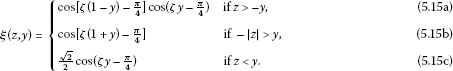Since

$cos\left[\zeta \left(1+y\right)-\frac{\pi }{4}\right]\ge cos\left(\zeta y+\frac{\pi }{4}\right)cos\left[\zeta \left(1+y\right)-\frac{\pi }{4}\right]>cos\left[\zeta \left(1-y\right)-\frac{\pi }{4}\right]cos\left(\zeta y-\frac{\pi }{4}\right),$

$\mathrm{\Phi }\left(y\right)=cos\left[\zeta \left(1+y\right)-\frac{\pi }{4}\right]$.

It follows, by studying the arguments of the cosines involved, that $-sin\left(\zeta \right)k\left(z,y\right)\le \frac{1}{2}\le \mathrm{\Phi }\left(y\right)$, therefore $sin\left(\zeta \right)|k\left(z,y\right)|\le \mathrm{\Phi }\left(y\right)$ for all $z,y\in \left[-1,1\right]$. □

We now give a technical lemma that will be used afterward.

Lemma 5.5 Let $f:\left[p-c,p+c\right]\to \mathbb{R}$ be a symmetric function with respect to p, decreasing in $\left[p,p+c\right]$. Let $g:\left[a,b\right]\to \mathbb{R}$ be an affine function such that $g\left(\left[a,b\right]\right)\subset \left[p-c,p+c\right]$. Under these hypotheses, the following hold:

1. (1)

If $g\left(a\right) or $p then $f\left(g\left(a\right)\right).

2. (2)

If $g\left(b\right) or $p then $f\left(g\left(a\right)\right)>f\left(g\left(b\right)\right)$.

3. (3)

If $g\left(a\right) then $f\left(g\left(a\right)\right) if and only if $g\left(\frac{a+b}{2}\right).

4. (4)

If $g\left(b\right) then $f\left(g\left(a\right)\right) if and only if $g\left(\frac{a+b}{2}\right)>p$.

Remark 5.6 An analogous result can be established, with the proper changes in the inequalities, if f is increasing in $\left[p,p+c\right]$.

Proof It is clear that $f\left(g\left(a\right)\right) if and only if $|g\left(a\right)-p|>|g\left(b\right)-p|$, so (1) and (2) are straightforward. Also, realize that, since g is affine, we have that $g\left(\frac{a+b}{2}\right)=\frac{g\left(a\right)+g\left(b\right)}{2}$.

Let us prove (3) as (4) is analogous:

$|g\left(b\right)-p|-|g\left(a\right)-p|=g\left(b\right)-p-\left(p-g\left(a\right)\right)=g\left(a\right)+g\left(b\right)-2p=2\left[g\left(\frac{a+b}{2}\right)-p\right].$

Therefore $|g\left(a\right)-p|>|g\left(b\right)-p|$ if and only if $g\left(\frac{a+b}{2}\right). □

Lemma 5.7 Let $\zeta \in \left(0,\frac{\pi }{4}\right]$ and $b\ge a\ge 0$ such that $a+b=1$. Then

$sin\left(\zeta \right)k\left(z,y\right)\ge c\left(a\right)\mathrm{\Phi }\left(y\right)\phantom{\rule{1em}{0ex}}\mathit{\text{for}}\phantom{\rule{0.5em}{0ex}}z\in \left[a,b\right],y\in \left[-1,1\right],$

where

$c\left(a\right):=\underset{y\in \left[-1,1\right]}{inf}\left\{\frac{sin\left(\zeta \right){inf}_{z\in \left[a,b\right]}k\left(z,y\right)}{\mathrm{\Phi }\left(y\right)}\right\}=\frac{\left[1-tan\left(\zeta a\right)\right]\left[1-tan\left(\zeta b\right)\right]}{\left[1+tan\left(\zeta a\right)\right]\left[1+tan\left(\zeta b\right)\right]}.$

Proof We know by Lemma 5.3 that k is positive in ${S}_{r}:=\left[a,b\right]×\left[-1,1\right]$. Furthermore, it is proved in  that

$\frac{\partial k}{\partial t}\left(t,s\right)+\omega k\left(-t,s\right)=0\phantom{\rule{1em}{0ex}}\mathrm{\forall }t,s\in \left[-T,T\right],$

so, differentiating and doing the proper substitutions we get that

$\frac{{\partial }^{2}k}{\partial {t}^{2}}\left(t,s\right)+{\omega }^{2}k\left(t,s\right)=0\phantom{\rule{1em}{0ex}}\mathrm{\forall }t,s\in \left[-T,T\right].$

Therefore, $\frac{{\partial }^{2}k}{\partial {t}^{2}}<0$ in ${S}_{r}$, which means that any minimum of k with respect to t has to be in the boundary of the differentiable regions of ${S}_{r}$. Thus, it is clear that, in ${S}_{r}$,(5.16)

By definition, $\eta \left(z,y\right)\ge \mathrm{\Psi }\left(y\right):=sin\left(\zeta \right){inf}_{z\in \left[a,b\right]}k\left(z,y\right)$. Also, realize that the arguments of the cosine in (5.7) are affine functions and that the cosine function is strictly decreasing in $\left[0,\pi \right]$ and symmetric with respect to zero. We can apply Lemma 5.5 to getFinally, we have to compare the cases (5.17b) with (5.17c) for $y\in \left[a,b\right)$ and (5.17d) with (5.17c) for $y\in \left[-b,-a\right)$. Using again Lemma 5.5, we obtain the following inequality:Thus, $\text{(5.17c)}>\text{(5.17b)}$ for $y\in \left[a,b\right)$.

To compare (5.17d) with (5.17c) for $y\in \left[-b,b\right)$ realize that k is continuous in the diagonal $z=-y$ (see ). Hence, since the expressions of (5.17d) and (5.17c) are already locally minimizing (in their differentiable components) for the variable z, it is clear that $\text{(5.17d)}\ge \text{(5.17c)}$ for $y\in \left[-b,-a\right)$. Therefore,It is easy to check that the following order holds:

$-1<-\frac{\pi }{4\zeta }<-b<\beta -1<1-\frac{\pi }{4\zeta }

Thus, we get the following expression:To find the infimum of this function, we will go through several steps in which we discard different cases. First, it is easy to check the inequalities $\text{(5.19g)}\ge \text{(5.19h)}=\text{(5.19a)}$ and $\text{(5.19d)}\ge \text{(5.19e)}$, so we need not to think about (5.19d), (5.19g) and (5.19h) anymore.

Now, realize that $|\zeta \left(1-b\right)-\frac{\pi }{4}|\le |\zeta b+\frac{\pi }{4}|\le \pi$. Since the cosine is decreasing in $\left[0,\pi \right]$ and symmetric with respect to zero this implies that $\text{(5.19e)}\ge \text{(5.19a)}$.

Note that (5.19c) can be written as

${g}_{1}\left(y\right):=\frac{\left[1-tan\left(\zeta y\right)\right]\left(1-tan\left[\zeta \left(1-y\right)\right]\right)}{\left[1+tan\left(\zeta y\right)\right]\left(1+tan\left[\zeta \left(1-y\right)\right]\right)}.$

Its derivative is

${g}_{1}^{\prime }\left(y\right)=-\frac{4\zeta \left[{tan}^{2}\left(\zeta y\right)-{tan}^{2}\zeta \left(y-1\right)\right]}{{\left(tan\zeta y+1\right)}^{2}{\left[tan\zeta \left(y-1\right)\right]}^{2}},$

which only vanishes at $y=\frac{1}{2}$ for $y\in \left[a,b\right]$.

${g}_{1}^{″}\left(\frac{1}{2}\right)=-\frac{16{\zeta }^{2}tan\left(\frac{\zeta }{2}\right)\left({tan}^{2}\frac{\zeta }{2}+1\right)}{{\left(tan\frac{\zeta }{2}+1\right)}^{4}}<0.$

Therefore, $y=\frac{1}{2}$ is a maximum of the function. Since ${g}_{1}$ is symmetric with respect to $\frac{1}{2}$ and a is the symmetric point of b with respect to $\frac{1}{2}$, $g\left(a\right)=g\left(b\right)$ is the infimum of (5.19c), which is contemplated in (5.19b) for $y=b$.

Making the change of variables $y=\overline{y}-1$, we have that (5.19f) can be written as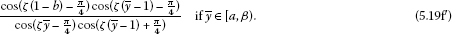Since $\text{(5.19e)}\ge \text{(5.19a)}$, it is clear now thatin $\left[b,\beta \right)$.

Let

${g}_{2}\left(y\right):=\frac{cos\left(\zeta \left(y-1\right)-\frac{\pi }{4}\right)}{cos\left(\zeta y-\frac{\pi }{4}\right)cos\left(\zeta \left(y-1\right)+\frac{\pi }{4}\right)}.$

Then

${g}_{2}^{\prime }\left(y\right)=\frac{\zeta }{4}\cdot \frac{sin\left[\zeta \left(2-y\right)-\frac{\pi }{4}\right]+sin\left[\zeta \left(3y-2\right)-\frac{\pi }{4}\right]+4cos\left[\zeta y-\frac{\pi }{4}\right]}{{sin}^{2}\left[\zeta y+\frac{\pi }{4}\right]{cos}^{2}{\left[\zeta \left(1-y\right)-\frac{\pi }{4}\right]}^{2}}.$

Since the argument in the cosine of the numerator is in the interval $\left[-\frac{\pi }{4},\frac{\pi }{4}\right]$ for $y\in \left[a,1\right]$, it is clear that ${g}_{2}^{\prime }\left(y\right)>0$ for $y\in \left[a,1\right]$, which implies that ${g}_{2}$ is increasing in that interval and (5.19b) and (5.19f) reach their infimum in the left extreme point of their intervals of definition.

We have then that

$\begin{array}{rcl}c\left(a\right)& =& \underset{y\in \left[-1,1\right]}{inf}\frac{\mathrm{\Psi }\left(y\right)}{\mathrm{\Phi }\left(y\right)}\\ =& min\left\{cos\left(\zeta b+\frac{\pi }{4}\right),\frac{cos\left(\zeta b+\frac{\pi }{4}\right)cos\left(\zeta \left(b-1\right)-\frac{\pi }{4}\right)}{cos\left(\zeta b-\frac{\pi }{4}\right)cos\left(\zeta \left(b-1\right)+\frac{\pi }{4}\right)},\frac{cos\left(-\zeta b-\frac{\pi }{4}\right)}{cos\left(-\zeta b+\frac{\pi }{4}\right)}\right\}.\end{array}$

The third element of the set is clearly greater or equal than the first. The second element is $cos\left(\zeta b+\frac{\pi }{4}\right){g}_{2}\left(b\right)$. Since ${g}_{2}$ is increasing in $\left[a,1\right]$,

$cos\left(\zeta b+\frac{\pi }{4}\right){g}_{2}\left(b\right)\le cos\left(\zeta b+\frac{\pi }{4}\right){g}_{2}\left(1\right)=cos\left(\zeta b+\frac{\pi }{4}\right)\frac{cos\left(\zeta \right)}{sin\left(\zeta \right)}\le cos\left(\zeta b+\frac{\pi }{4}\right).$

Therefore,

$c\left(a\right)=\frac{cos\left(\zeta b+\frac{\pi }{4}\right)cos\left(\zeta \left(b-1\right)-\frac{\pi }{4}\right)}{cos\left(\zeta b-\frac{\pi }{4}\right)cos\left(\zeta \left(b-1\right)+\frac{\pi }{4}\right)}=\frac{\left[1-tan\left(\zeta a\right)\right]\left[1-tan\left(\zeta b\right)\right]}{\left[1+tan\left(\zeta a\right)\right]\left[1+tan\left(\zeta b\right)\right]}.$

□

Remark 5.8 It is easy to find an upper estimate of $c\left(a\right)$. Just assume $a=b=\frac{1}{2}$.

$c\left(a\right)\le c\left(0\right)={\left(\frac{1-tan\frac{\zeta }{2}}{1+tan\frac{\zeta }{2}}\right)}^{2}\le {\left(\frac{1-tan\frac{\pi }{8}}{1+tan\frac{\pi }{8}}\right)}^{2}=\frac{{\left(2-\sqrt{2}\right)}^{2}}{2}=0.17157\dots .$

We can do the same study for $\zeta \in \left(0,\frac{\pi }{4}\right]$. The proofs are almost the same, but in this case the calculations are much easier.

Lemma 5.9 If $\zeta \in \left(0,\frac{\pi }{4}\right]$ then $sin\left(\zeta \right)|k\left(z,y\right)|\le \mathrm{\Phi }\left(y\right):={max}_{z\in \left[-1,1\right]}k\left(z,y\right)$ where Φ admits the following expression:

$\mathrm{\Phi }\left(y\right)=\left\{\begin{array}{cc}cos\left[\zeta \left(y-1\right)+\frac{\pi }{4}\right]cos\left(\zeta y-\frac{\pi }{4}\right)\hfill & \mathit{\text{if}}\phantom{\rule{0.5em}{0ex}}y\in \left[0,1\right],\hfill \\ cos\left(\zeta y+\frac{\pi }{4}\right)cos\left[\zeta \left(y+1\right)-\frac{\pi }{4}\right]\hfill & \mathit{\text{if}}\phantom{\rule{0.5em}{0ex}}y\in \left[-1,0\right).\hfill \end{array}$

Proof This time, a simplified version of inequality (5.9) holds,

(5.20)

so we only need to study two cases. If $y>0$, we are in the same situation as in the case $y\in \left[1-\frac{\pi }{4\zeta },\frac{\pi }{4\zeta }\right)$ studied in Lemma 5.4. Hence, $\mathrm{\Phi }\left(y\right)=cos\left[\zeta \left(y-1\right)+\frac{\pi }{4}\right]cos\left(\zeta y-\frac{\pi }{4}\right)$. If $y<0$ we are in the same situation as in the case $y\in \left[-\frac{\pi }{4\zeta },\frac{\pi }{4\zeta }-1\right)$. Therefore, $\mathrm{\Phi }\left(y\right)=cos\left(\zeta y+\frac{\pi }{4}\right)cos\left[\zeta \left(y+1\right)-\frac{\pi }{4}\right]$. □

Lemma 5.10 Let $\zeta \in \left(0,\frac{\pi }{4}\right]$ and $b\ge a\ge 0$ such that $a+b=1$. Then

$sin\left(\zeta \right)k\left(z,y\right)\ge c\left(a\right)\mathrm{\Phi }\left(y\right)\phantom{\rule{1em}{0ex}}\mathit{\text{for}}\phantom{\rule{0.5em}{0ex}}z\in \left[a,b\right],y\in \left[-1,1\right],$

where

$c\left(a\right):=\underset{y\in \left[-1,1\right]}{inf}\left\{\frac{sin\left(\zeta \right){inf}_{z\in \left[a,b\right]}k\left(z,y\right)}{\mathrm{\Phi }\left(y\right)}\right\}=\frac{\left[1-tan\left(\zeta a\right)\right]\left[1-tan\left(\zeta b\right)\right]}{\left[1+tan\left(\zeta a\right)\right]\left[1+tan\left(\zeta b\right)\right]}.$

Proof Ψ is as in (5.18a)-(5.18d), but we get the simpler expressionBy the same kind of arguments used in the proof of Lemma 5.7, we get the desired result. □

Lemma 5.11

$\underset{t\in \left[-T,T\right]}{sup}{\int }_{-T}^{T}|k\left(t,s\right)|\phantom{\rule{0.2em}{0ex}}ds=\left\{\begin{array}{cc}\frac{1}{\omega }\hfill & \mathit{\text{if}}\phantom{\rule{0.5em}{0ex}}\zeta \in \left(0,\frac{\pi }{4}\right],\hfill \\ \frac{1}{\omega }\left[1+\frac{\sqrt{2}cos\frac{2\zeta +\pi }{3}sin\frac{\pi -4\zeta }{12}+cos\frac{\pi -\zeta }{3}\left(1-sin\frac{2\zeta +\pi }{3}\right)}{sin\zeta }\right]\hfill & \mathit{\text{if}}\phantom{\rule{0.5em}{0ex}}\zeta \in \left[\frac{\pi }{4},\frac{\pi }{2}\right].\hfill \end{array}$

Proof First of all, if $\zeta \in \left[0,\frac{\pi }{4}\right]$, then $|k\left(t,s\right)|=k\left(t,s\right)$. The solution of the problem ${x}^{\prime }\left(t\right)+\omega x\left(-t\right)=1$, $x\left(-T\right)=x\left(T\right)$ is clearly $u\left(t\right)\equiv \frac{1}{\omega }$, but at the same time it has to be of the kind in equation (5.5), so $u\left(t\right)={\int }_{-T}^{T}k\left(t,s\right)\phantom{\rule{0.2em}{0ex}}ds$. This proves the first part.

If $\zeta \in \left[\frac{\pi }{4},\frac{\pi }{2}\right]$, then

${\int }_{-T}^{T}|k\left(t,s\right)|\phantom{\rule{0.2em}{0ex}}ds={\int }_{-T}^{T}{k}^{+}\left(t,s\right)\phantom{\rule{0.2em}{0ex}}ds+{\int }_{-T}^{T}{k}^{-}\left(t,s\right)\phantom{\rule{0.2em}{0ex}}ds=\frac{1}{\omega }+2{\int }_{-T}^{T}{k}^{-}\left(t,s\right)\phantom{\rule{0.2em}{0ex}}ds.$

We make two observations here.

From equation (5.6), it follows that $k\left(t+T,s+T\right)=k\left(t,s\right)$ and $k\left(t+T,s\right)=k\left(t,s+T\right)$ for a.e. $t,s\in \left[-T,0\right]$. Hence, for $t\in \left[-T,0\right]$ and a function $\xi :\mathbb{R}\to \mathbb{R}$, using the change of variables $r=s+T$, $\tau =s-T$, we have that

$\begin{array}{rcl}{\int }_{-T}^{T}\xi \left(k\left(t+T,s\right)\right)\phantom{\rule{0.2em}{0ex}}ds& =& {\int }_{-T}^{0}\xi \left(k\left(t+T,s\right)\right)\phantom{\rule{0.2em}{0ex}}ds+{\int }_{0}^{T}\xi \left(k\left(t+T,s\right)\right)\phantom{\rule{0.2em}{0ex}}ds\\ =& {\int }_{-T}^{0}\xi \left(k\left(t,s+T\right)\right)\phantom{\rule{0.2em}{0ex}}ds+{\int }_{-T}^{0}\xi \left(k\left(t+T,\tau +T\right)\right)\phantom{\rule{0.2em}{0ex}}d\tau \\ =& {\int }_{0}^{T}\xi \left(k\left(t,r\right)\right)\phantom{\rule{0.2em}{0ex}}dr+{\int }_{-T}^{0}\xi \left(k\left(t,\tau \right)\right)\phantom{\rule{0.2em}{0ex}}d\tau \\ =& {\int }_{-T}^{T}\xi \left(k\left(t,s\right)\right)\phantom{\rule{0.2em}{0ex}}ds.\end{array}$

Therefore, ${sup}_{t\in \left[-T,T\right]}{\int }_{-T}^{T}|k\left(t,s\right)|\phantom{\rule{0.2em}{0ex}}ds={sup}_{t\in \left[-T,0\right]}{\int }_{-T}^{T}|k\left(t,s\right)|\phantom{\rule{0.2em}{0ex}}ds$. The second observation is that, taking into account Lemma 5.3, $k\left(t,s\right)$ is positive in $\left(-\frac{\pi }{4\omega },1-\frac{\pi }{4\omega }\right)×\left[-1,1\right]$, so

$\underset{t\in \left[-T,0\right]}{sup}{\int }_{-T}^{T}|k\left(t,s\right)|\phantom{\rule{0.2em}{0ex}}ds=\underset{t\in \left[-T,0\right]\setminus \left(-\frac{\pi }{4\omega },1-\frac{\pi }{4\omega }\right)}{sup}{\int }_{-T}^{T}|k\left(t,s\right)|\phantom{\rule{0.2em}{0ex}}ds.$

Using the same kind of arguments as in Lemma 5.3, it follows that $k\left(t,s\right)$ is negative in $\left(-T,-\frac{\pi }{4\omega }\right)×\left(t,-\frac{\pi }{4\omega }\right)$ if $t\in \left(-T,-\frac{\pi }{4\omega }\right)$ and $\left(\frac{\pi }{4\omega }-1,0\right)×\left(t,1-\frac{\pi }{4\omega }\right)$ if $t\in \left(\frac{\pi }{4\omega }-1,0\right)$, so it is enough to compute $\eta \left(t\right):={\int }_{B\left(t\right)}{k}^{-}\left(t,s\right)\phantom{\rule{0.2em}{0ex}}ds$ where $B\left(t\right)=\left\{s\in \left[-T,T\right]:\left(t,s\right)\in supp\left({k}^{-}\right)\right\}$.

With the change of variable $t=zT$,

where

${\eta }_{1}\left(z\right)=cos\left[\zeta \left(z+1\right)+\frac{\pi }{4}\right]\left[1+sin\left(\zeta z-\frac{\pi }{4}\right)\right]$

and

${\eta }_{2}\left(z\right)=\sqrt{2}cos\left[\zeta \left(z+1\right)+\frac{\pi }{4}\right]sin\zeta z+cos\left(\zeta z+\frac{\pi }{4}\right)\left[1-sin\left(\zeta \left(z+1\right)+\frac{\pi }{4}\right)\right].$

It follows thatWith these facts, we conclude that there is a unique maximum of the function $\eta \left(z\right)$ in the interval $\left(\frac{\pi }{4\zeta }-1,0\right)$, precisely where ${\eta }_{2}^{\prime }\left(z\right)=\zeta \left(cos\left[\zeta \left(1+2z\right)\right]-sin\left(\frac{\pi }{4}+z\zeta \right)\right)=0$, this is, for $z=\frac{1}{3}\left(\frac{\pi }{4}-1\right)$ and, therefore, the statement of the theorem holds. □

Lemma 5.12 Let $\omega \in \left[\frac{\pi }{4}T,\frac{\pi }{2}T\right]$ and $T-\frac{\pi }{4\omega }. Then

$2\omega sin\left(\zeta \right)\underset{t\in \left[a,b\right]}{inf}{\int }_{a}^{b}k\left(t,s\right)\phantom{\rule{0.2em}{0ex}}ds=sin\omega \left(T-2a\right)+cos\zeta -cos2\omega a.$

Proof It follows thatTherefore, ${\int }_{a}^{b}k\left(t,s\right)\phantom{\rule{0.2em}{0ex}}ds={\int }_{-T}^{b}k\left(t,s\right)\phantom{\rule{0.2em}{0ex}}ds-{\int }_{-T}^{a}k\left(t,s\right)\phantom{\rule{0.2em}{0ex}}ds$, this is,Using similar arguments to the ones used in the proof of Lemma 5.7, we can show that

$2\omega sin\left(\zeta \right)\underset{t\in \left[a,b\right]}{inf}{\int }_{a}^{b}k\left(t,s\right)\phantom{\rule{0.2em}{0ex}}ds=sin\omega \left(T-2a\right)+cos\zeta -cos2\omega a.$

□

With the same method, we can prove the following corollary.

Corollary 5.13 Let $\omega \in \left(0,\frac{\pi }{4}T\right]$ and $0. Then

$2\omega sin\left(\zeta \right)\underset{t\in \left[a,b\right]}{inf}{\int }_{a}^{b}k\left(t,s\right)\phantom{\rule{0.2em}{0ex}}ds=sin\omega \left(T-2a\right)+cos\zeta -cos2\omega a.$

Remark 5.14 If $\omega \in \left(0,\frac{\pi }{4}T\right]$, then

$\underset{t\in \left[-T,T\right]}{inf}{\int }_{-T}^{T}k\left(t,s\right)\phantom{\rule{0.2em}{0ex}}ds=\frac{1}{\omega },$

just because of the observation in the proof of Lemma 5.11.

Now we can state conditions (${I}_{\rho }^{0}$) and (${I}_{\rho }^{1}$) for the special case of problem (5.1)-(5.2):

(${\mathrm{I}}_{\rho ,\omega }^{1}$) Let

${f}_{\omega }^{-\rho ,\rho }:=sup\left\{\frac{h\left(t,u,v\right)+\omega v}{\rho }:\left(t,u,v\right)\in \left[-T,T\right]×\left[-\rho ,\rho \right]×\left[-\rho ,\rho \right]\right\}.$

There exist $\rho >0$ and $\omega \in \left(0,\frac{\pi }{4}\right]$ such that ${f}_{\omega }^{-\rho ,\rho }<\omega$,ORthere exist $\rho >0$ and $\omega \in \left(\frac{\pi }{4},\frac{\pi }{2}\right]$ such that

${f}_{\omega }^{-\rho ,\rho }\cdot \left[1+\frac{\sqrt{2}cos\frac{2\zeta +\pi }{3}sin\frac{\pi -4\zeta }{12}+cos\frac{\pi -\zeta }{3}\left(1-sin\frac{2\zeta +\pi }{3}\right)}{sin\zeta }\right]<\omega .$

(${\mathrm{I}}_{\rho ,\omega }^{0}$) There exist $\rho >0$ such that such that

${f}_{\left(\rho ,\rho /c\right)}^{\omega }\cdot \underset{t\in \left[a,b\right]}{inf}{\int }_{a}^{b}k\left(t,s\right)\phantom{\rule{0.2em}{0ex}}ds>1,$

where

${f}_{\left(\rho ,\rho /c\right)}^{\omega }=inf\left\{\frac{h\left(t,u,v\right)+\omega v}{\rho }:\left(t,u,v\right)\in \left[a,b\right]×\left[\rho ,\rho /c\right]×\left[-\rho /c,\rho /c\right]\right\}.$

Theorem 5.15 Let $\omega \in \left(0,\frac{\pi }{2}T\right]$. Let $\left[a,b\right]\subset \left[-T,T\right]$ such that $a=1-b\in \left(max\left\{0,T-\frac{\pi }{4\omega }\right\},\frac{T}{2}\right)$. Let

$c=\frac{\left[1-tan\left(\omega a\right)\right]\left[1-tan\left(\omega b\right)\right]}{\left[1+tan\left(\omega a\right)\right]\left[1+tan\left(\omega b\right)\right]}.$

Problem (5.1)-(5.2) has at least one nonzero solution in K if either of the following conditions hold:

(S1) There exist ${\rho }_{1},{\rho }_{2}\in \left(0,\mathrm{\infty }\right)$ with ${\rho }_{1}/c<{\rho }_{2}$ such that (${\mathrm{I}}_{{\rho }_{1},\omega }^{0}$) and (${\mathrm{I}}_{{\rho }_{2},\omega }^{1}$) hold.

(S2) There exist ${\rho }_{1},{\rho }_{2}\in \left(0,\mathrm{\infty }\right)$ with ${\rho }_{1}<{\rho }_{2}$ such that (${\mathrm{I}}_{{\rho }_{1},\omega }^{1}$) and (${\mathrm{I}}_{{\rho }_{2},\omega }^{0}$) hold.

The integral equation (2.1) has at least two nonzero solutions in K if one of the following conditions hold:

(S3) There exist ${\rho }_{1},{\rho }_{2},{\rho }_{3}\in \left(0,\mathrm{\infty }\right)$ with ${\rho }_{1}/c<{\rho }_{2}<{\rho }_{3}$ such that (${\mathrm{I}}_{{\rho }_{1},\omega }^{0}$), (${\mathrm{I}}_{{\rho }_{2},\omega }^{1}$) and (${\mathrm{I}}_{{\rho }_{3},\omega }^{0}$) hold.

(S4) There exist ${\rho }_{1},{\rho }_{2},{\rho }_{3}\in \left(0,\mathrm{\infty }\right)$ with ${\rho }_{1}<{\rho }_{2}$ and ${\rho }_{2}/c<{\rho }_{3}$ such that (${\mathrm{I}}_{{\rho }_{1},\omega }^{1}$), (${\mathrm{I}}_{{\rho }_{2},\omega }^{0}$) and (${\mathrm{I}}_{{\rho }_{3},\omega }^{1}$) hold.

The integral equation (2.1) has at least three nonzero solutions in K if one of the following conditions hold:

(S5) There exist ${\rho }_{1},{\rho }_{2},{\rho }_{3},{\rho }_{4}\in \left(0,\mathrm{\infty }\right)$ with ${\rho }_{1}/c<{\rho }_{2}<{\rho }_{3}$ and ${\rho }_{3}/c<{\rho }_{4}$ such that (${\mathrm{I}}_{{\rho }_{1},\omega }^{0}$), (${\mathrm{I}}_{{\rho }_{2},\omega }^{1}$), (${\mathrm{I}}_{{\rho }_{3},\omega }^{0}$) and (${\mathrm{I}}_{{\rho }_{4},\omega }^{1}$) hold.

(S6) There exist ${\rho }_{1},{\rho }_{2},{\rho }_{3},{\rho }_{4}\in \left(0,\mathrm{\infty }\right)$ with ${\rho }_{1}<{\rho }_{2}$ and ${\rho }_{2}/c<{\rho }_{3}<{\rho }_{4}$ such that (${\mathrm{I}}_{{\rho }_{1},\omega }^{1}$), (${\mathrm{I}}_{{\rho }_{2},\omega }^{0}$), (${\mathrm{I}}_{{\rho }_{3},\omega }^{1}$) and (${\mathrm{I}}_{{\rho }_{4},\omega }^{0}$) hold.

### 5.1 Example

Consider problem (5.1)-(5.2) with

$h\left(t,u,v\right)=\frac{1}{2+{\left(t-1\right)}^{2}}+\frac{{u}^{2}}{5}+2u+\frac{1}{1+7{v}^{2}}+7.$

Let $T=1$, $\zeta =\omega =1.5$, $a=0.48$, $b=0.52$, ${\rho }_{1}=1$, ${\rho }_{2}=2$. Conditions (C1)-(C3) are clearly satisfied by the results proved before. (C4) follows the expression of h, so we are in the hypothesis of Theorem 2.4. Also,Clearly, ${f}_{\omega }^{-{\rho }_{1},{\rho }_{1}}<{r}_{1}$ and ${f}_{\left({\rho }_{2},{\rho }_{2}/c\right)}^{\omega }>{r}_{2}$, so condition (S2) in the previous theorem is satisfied and, therefore, the problem (5.1)-(5.2) has at least one solution.

## References

1. Cabada A, Tojo FAF: Comparison results for first order linear operators with reflection and periodic boundary value conditions. Nonlinear Anal. 2013, 78: 32-46.

2. Cabada A, Cid JA: On comparison principles for the periodic Hill’s equation. J. Lond. Math. Soc. 2012, 86: 272-290. 10.1112/jlms/jds001

3. Cabada A, Cid JA, Máquez-Villamarín B: Computation of Green’s functions for boundary value problems with Mathematica. Appl. Math. Comput. 2012, 219: 1919-1936. 10.1016/j.amc.2012.08.035

4. Aftabizadeh AR, Huang YK, Wiener J: Bounded solutions for differential equations with reflection of the argument. J. Math. Anal. Appl. 1988, 135: 31-37. 10.1016/0022-247X(88)90139-4

5. Andrade D, Ma TF: Numerical solutions for a nonlocal equation with reflection of the argument. Neural Parallel Sci. Comput. 2002, 10: 227-233.

6. Gupta CP: Existence and uniqueness theorems for boundary value problems involving reflection of the argument. Nonlinear Anal. 1987, 11: 1075-1083. 10.1016/0362-546X(87)90085-X

7. Gupta CP: Two-point boundary value problems involving reflection of the argument. Int. J. Math. Math. Sci. 1987, 10: 361-371. 10.1155/S0161171287000425

8. Ma TF, Miranda ES, de Souza Cortes MB: A nonlinear differential equation involving reflection of the argument. Arch. Math. 2004, 40: 63-68.

9. O’Regan D: Existence results for differential equations with reflection of the argument. J. Aust. Math. Soc. A 1994, 57: 237-260. 10.1017/S1446788700037538

10. O’Regan D, Zima M: Leggett-Williams norm-type fixed point theorems for multivalued mappings. Appl. Math. Comput. 2007, 187: 1238-1249. 10.1016/j.amc.2006.09.035

11. Piao D: Pseudo almost periodic solutions for differential equations involving reflection of the argument. J. Korean Math. Soc. 2004, 41: 747-754.

12. Piao D: Periodic and almost periodic solutions for differential equations with reflection of the argument. Nonlinear Anal. 2004, 57: 633-637. 10.1016/j.na.2004.03.017

13. Wiener J, Aftabizadeh AR: Boundary value problems for differential equations with reflection of the argument. Int. J. Math. Math. Sci. 1985, 8: 151-163. 10.1155/S016117128500014X

14. Torres PJ: Existence of one-signed periodic solutions of some second-order differential equations via a Krasnoselskii fixed point theorem. J. Differ. Equ. 2003, 190: 643-662. 10.1016/S0022-0396(02)00152-3

15. Guo D, Lakshmikantham V: Nonlinear Problems in Abstract Cones. Academic Press, Boston; 1988.

16. Infante G, Webb JRL: Three point boundary value problems with solutions that change sign. J. Integral Equ. Appl. 2003, 15: 37-57. 10.1216/jiea/1181074944

17. Infante G, Webb JRL: Nonzero solutions of Hammerstein integral equations with discontinuous kernels. J. Math. Anal. Appl. 2002, 272: 30-42. 10.1016/S0022-247X(02)00125-7

18. Amann H: Fixed point equations and nonlinear eigenvalue problems in ordered Banach spaces. SIAM Rev. 1976, 18: 620-709. 10.1137/1018114

19. Franco D, Infante G, O’Regan D: Positive and nontrivial solutions for the Urysohn integral equation. Acta Math. Sin. 2006, 22: 1745-1750. 10.1007/s10114-005-0782-3

20. Franco D, Infante G, O’Regan D: Nontrivial solutions in abstract cones for Hammerstein integral systems. Dyn. Contin. Discrete Impuls. Syst., Ser. A, Math. Anal. 2007, 14: 837-850.

21. Fan H, Ma R: Loss of positivity in a nonlinear second order ordinary differential equations. Nonlinear Anal. 2009, 71: 437-444. 10.1016/j.na.2008.10.117

22. Infante G: Eigenvalues of some non-local boundary-value problems. Proc. Edinb. Math. Soc. 2003, 46: 75-86.

23. Infante G, Pietramala P: Nonlocal impulsive boundary value problems with solutions that change sign. 1124. In Mathematical Models in Engineering, Biology and Medicine: Proceedings of the International Conference on Boundary Value Problems Edited by: Cabada A, Liz E, Nieto JJ. 2009, 205-213.

24. Infante G, Pietramala P: Perturbed Hammerstein integral inclusions with solutions that change sign. Comment. Math. Univ. Carol. 2009, 50: 591-605.

25. Infante G, Webb JRL: Loss of positivity in a nonlinear scalar heat equation. Nonlinear Differ. Equ. Appl. 2006, 13: 249-261. 10.1007/s00030-005-0039-y

26. Infante G, Webb JRL: Nonlinear nonlocal boundary value problems and perturbed Hammerstein integral equations. Proc. Edinb. Math. Soc. 2006, 49: 637-656. 10.1017/S0013091505000532

27. Nieto JJ, Pimentel J: Positive solutions of a fractional thermostat model. Bound. Value Probl. 2013., 2013: Article ID 5

28. Krasnosel’skiĭ MA, Zabreĭko PP: Geometrical Methods of Nonlinear Analysis. Springer, Berlin; 1984.

29. Martin RH: Nonlinear Operators and Differential Equations in Banach Spaces. Wiley, New York; 1976.

30. Lan KQ: Multiple positive solutions of Hammerstein integral equations with singularities. Differ. Equ. Dyn. Syst. 2000, 8: 175-195.

31. Lan KQ: Multiple positive solutions of Hammerstein integral equations and applications to periodic boundary value problems. Appl. Math. Comput. 2004, 154: 531-542. 10.1016/S0096-3003(03)00733-1

32. Infante G: Positive solutions of some nonlinear BVPs involving singularities and integral BCs. Discrete Contin. Dyn. Syst., Ser. S 2008, 1: 99-106.

33. Infante G: Positive solutions of nonlocal boundary value problems with singularities. Discrete Contin. Dyn. Syst. 2009, 2009: 377-384. suppl.

34. Infante, G, Pietramala, P: The displacement of a sliding bar subject to nonlinear controllers. In: Proceedings of the International Conference on Differential & Difference Equations and Applications. Springer, Berlin (in press)

35. Webb JRL: A class of positive linear operators and applications to nonlinear boundary value problems. Topol. Methods Nonlinear Anal. 2012, 39: 221-242.

36. Webb JRL, Zima M: Multiple positive solutions of resonant and non-resonant nonlocal boundary value problems. Nonlinear Anal. 2009, 71: 1369-1378. 10.1016/j.na.2008.12.010

## Acknowledgements

It is a great pleasure for us to dedicate this paper to Professor Jean Mawhin on the occasion of his seventieth birthday.

A. Cabada was partially supported by FEDER and Ministerio de Educación y Ciencia, Spain, project MTM2010-15314. This paper was partially written during the visit of G. Infante to the Departamento de Análise Matemática of the Universidade de Santiago de Compostela. G. Infante is grateful to the people of the aforementioned Departamento for their kind and warm hospitality. F.A.F. Tojo was partially supported by Diputación de A Coruña, Bolsas para Investigación 2012.

## Author information

Authors

### Competing interests

The authors declare that they have no competing interests.

### Authors’ contributions

The three authors have participated into the obtained results. The collaboration of each one cannot be separated in different parts of the paper. All of them have made substantial contributions to the theoretical results. The three authors have been involved in drafting the manuscript and revising it critically for important intellectual content. All authors have given final approval of the version to be published.

## Rights and permissions

Reprints and Permissions

Cabada, A., Infante, G. & Fernández Tojo, F.A. Nontrivial solutions of Hammerstein integral equations with reflections. Bound Value Probl 2013, 86 (2013). https://doi.org/10.1186/1687-2770-2013-86